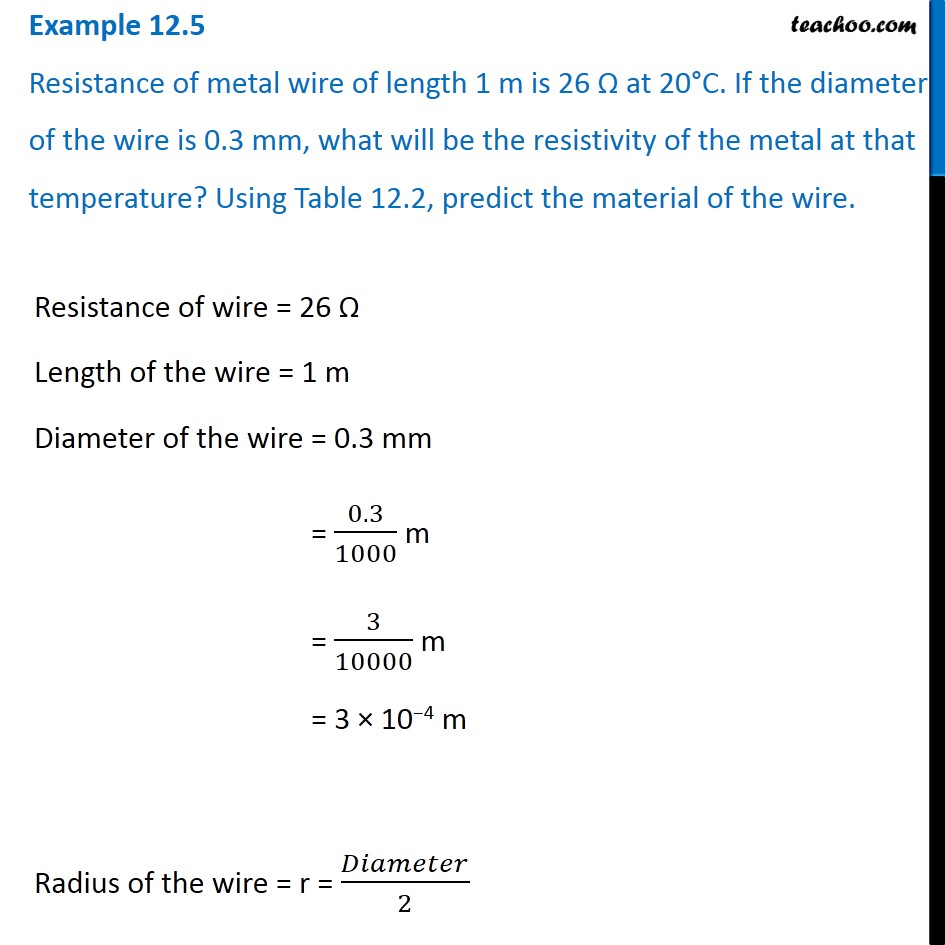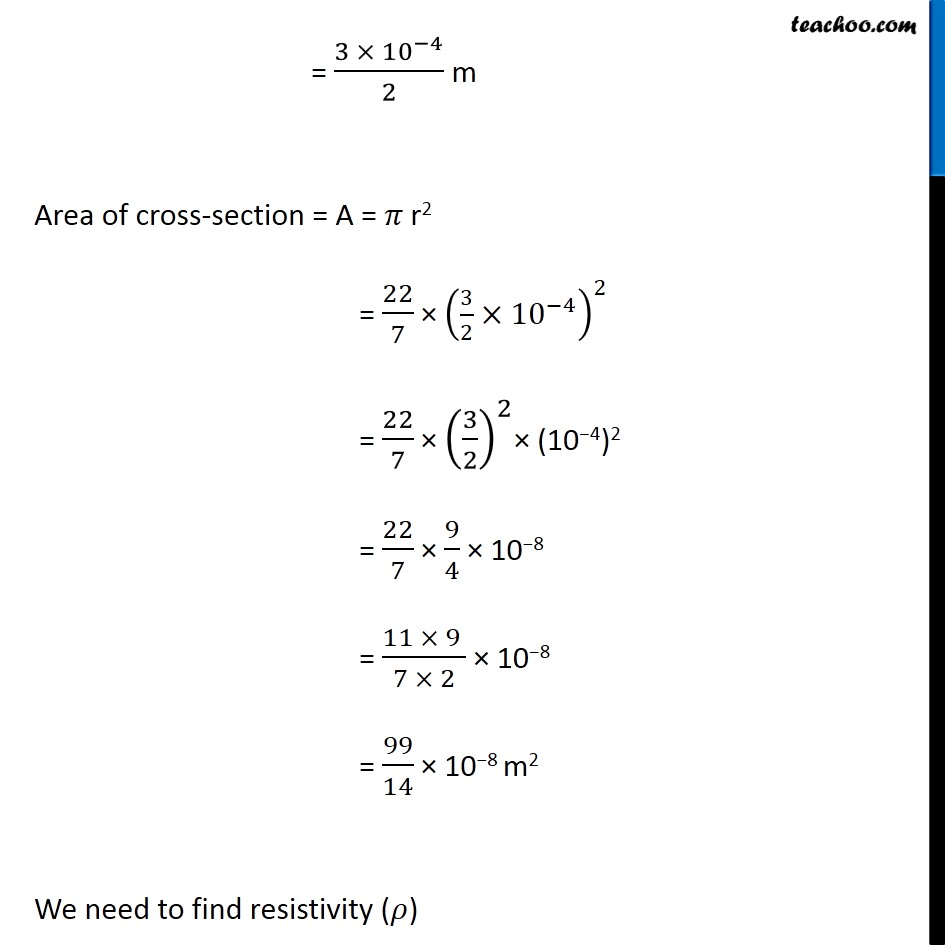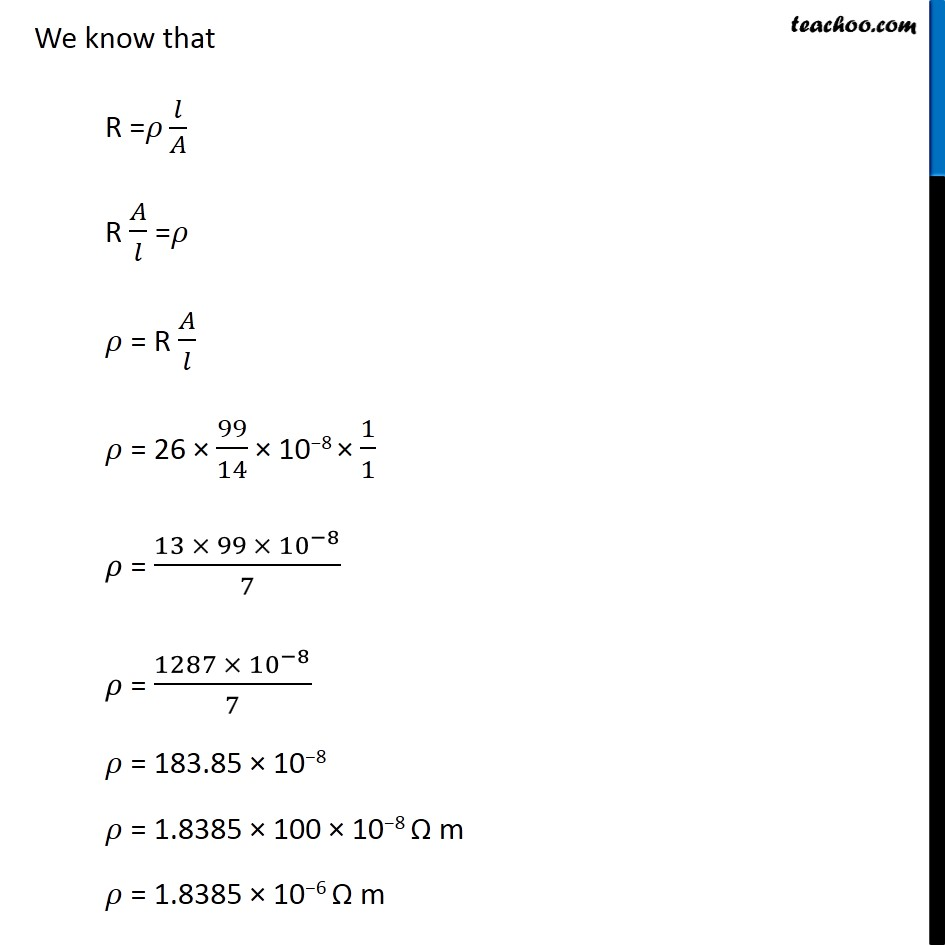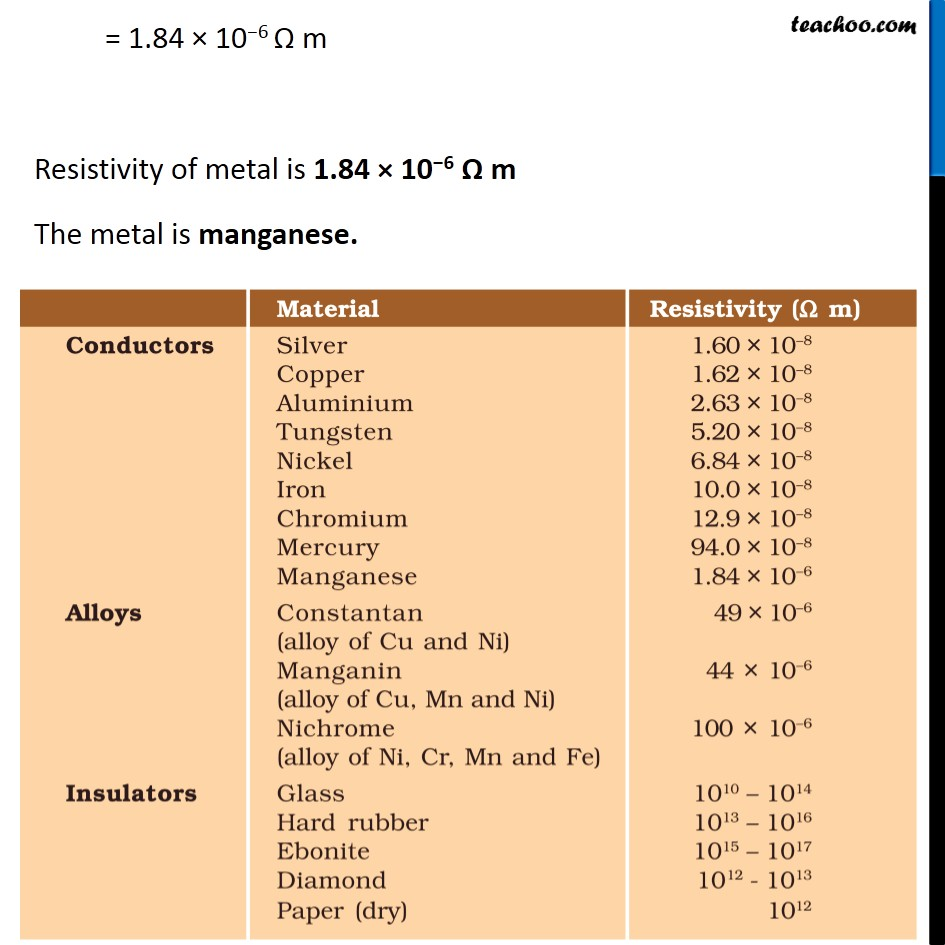Examples from NCERT Book

Class 10
Chapter 12 Class 10 - Electricity

## Resistance of metal wire of length 1 m is 26 Ω at 20°C. If the diameter of the wire is 0.3 mm, what will be the resistivity of the metal at that temperature? Using Table 12.2, predict the material of the wire.Learn in your speed, with individual attention - Teachoo Maths 1-on-1 Class

### Transcript

Example 12.5 Resistance of metal wire of length 1 m is 26 Ω at 20°C. If the diameter of the wire is 0.3 mm, what will be the resistivity of the metal at that temperature? Using Table 12.2, predict the material of the wire. Resistance of wire = 26 Ω Length of the wire = 1 m Diameter of the wire = 0.3 mm = 0.3/1000 m = 3/10000 m = 3 × 10−4 m Radius of the wire = r = 𝐷𝑖𝑎𝑚𝑒𝑡𝑒𝑟/2 = (3 × 〖10〗^(−4))/2 m Area of cross-section = A = 𝜋 r2 = 22/7 × (3/2×〖10〗^(−4) )^2 = 22/7 × (3/2)^2× (10−4)2 = 22/7 × 9/4 × 10−8 = (11 × 9 )/(7 × 2) × 10−8 = 99/14 × 10−8 m2 We need to find resistivity (𝜌) We know that R =𝜌 𝑙/𝐴 R 𝐴/𝑙 =𝜌 𝜌 = R 𝐴/𝑙 𝜌 = 26 × 99/14 × 10−8 × 1/1 𝜌 = (13 × 99 × 〖10〗^(−8))/7 𝜌 = (1287 × 〖10〗^(−8))/7 𝜌 = 183.85 × 10−8 𝜌 = 1.8385 × 100 × 10−8 Ω m 𝜌 = 1.8385 × 10−6 Ω m = 1.84 × 10−6 Ω m Resistivity of metal is 1.84 × 10−6 Ω m The metal is manganese.